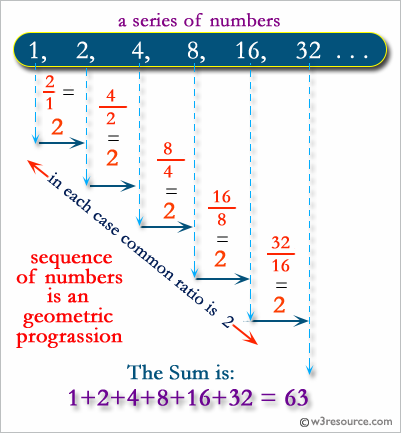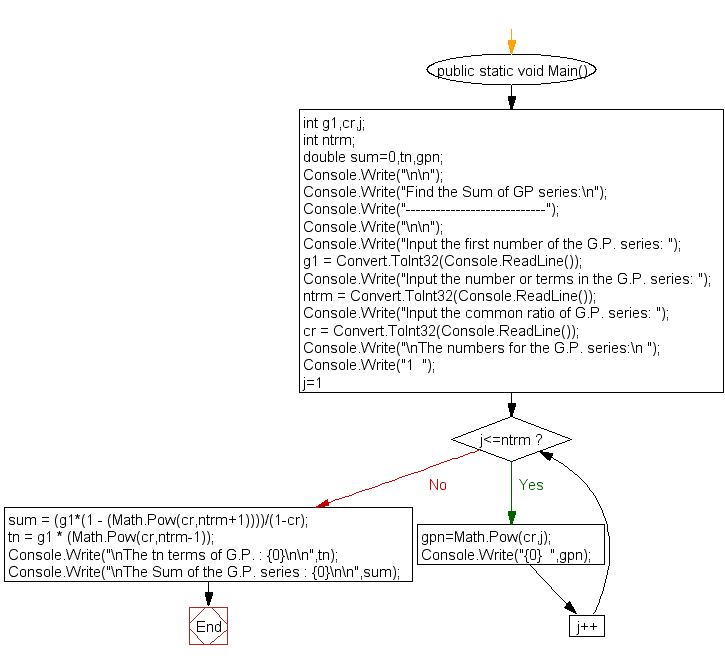﻿ C# - Find the Sum of GP series - w3resource# C# Sharp Exercises: Find the Sum of GP series

## C# Sharp For Loop: Exercise-52 with Solution

Write a program in C# Sharp to find the Sum of GP series.Sample Solution:-

C# Sharp Code:

``````using System;
public class Exercise52
{
public static void Main()
{

int g1,cr,j;
int ntrm;
double sum=0,tn,gpn;

Console.Write("\n\n");
Console.Write("Find the Sum of GP series:\n");
Console.Write("----------------------------");
Console.Write("\n\n");

Console.Write("Input the first number of the G.P. series: ");

Console.Write("Input the number or terms in the G.P. series: ");

Console.Write("Input the common ratio of G.P. series: ");

/*-------- generate G.P. series ---------------*/
Console.Write("\nThe numbers for the G.P. series:\n ");
Console.Write("1  ");

for(j=1;j<=ntrm;j++)
{
gpn=Math.Pow(cr,j);
Console.Write("{0}  ",gpn);
}
/*-------- End of G.P. series generate ---------------*/

sum = (g1*(1 - (Math.Pow(cr,ntrm+1))))/(1-cr);
tn = g1 * (Math.Pow(cr,ntrm-1));

Console.Write("\nThe tn terms of G.P. : {0}\n\n",tn);
Console.Write("\nThe Sum of the G.P. series : {0}\n\n",sum);
}
}
```
```

Sample Output:

```Find the Sum of GP series:
----------------------------
Input the first number of the G.P. series: 1
Input the number or terms in the G.P. series: 5
Input the common ratio of G.P. series: 10
The numbers for the G.P. series:
1  10  100  1000  10000  100000
The tn terms of G.P. : 10000
The Sum of the G.P. series : 111111
```

Flowchart:C# Sharp Code Editor:

Contribute your code and comments through Disqus.

What is the difficulty level of this exercise?

Test your Programming skills with w3resource's quiz.

﻿